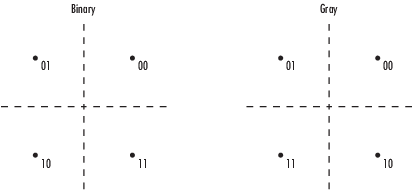# QPSK Modulator Baseband

Modulate using quadrature phase shift keying method

## Library

PM in Digital Baseband sublibrary of Modulation

•## Description

The QPSK Modulator Baseband block modulates using the quadrature phase shift keying method. The output is a baseband representation of the modulated signal.

### Integer-Valued Signals and Binary-Valued Signals

If you set the Input type parameter to `Integer`, then valid input values are 0, 1, 2, and 3. When you set Constellation ordering to `Binary` for input m the output symbol is

exp(jθ + jπm/2)

where θ represents the Phase offset parameter (see the following figure for Gray constellation ordering). In this case, the block accepts a scalar or column vector signal.

If you set the Input type parameter to `Bit`, then the input contains pairs of binary values. For this configuration, the block accepts column vectors with even lengths. When you set the Phase offset parameter to $\frac{\Pi }{4}$, then the block uses one of the signal constellations in the following figure, depending on whether you set the Constellation ordering parameter to `Binary` or `Gray`.In the previous figure, the most significant bit (i.e. the left-most bit), is the first bit input to the block. For additional information about Gray mapping, see the M-PSK Modulator Baseband help page.

## Dialog Box

Input type

Indicates whether the input consists of integers or pairs of bits.

Constellation ordering

Determines how the block maps each pair of input bits or input integers to constellation symbols.

The phase of the zeroth point of the signal constellation.

View Constellation

Click on the block mask to visualize a signal constellation for the specified block parameters. Before viewing a constellation, apply the parameter settings. For more information, see View Constellation of Modulator Block.

Output data type

Output data type, specified as `double`, `single`, `fixdt(1,16)`, `fixdt(1,16, 0)`, ```Inherit via back propagation```, or ```<data type expression>```.

Setting this parameter to

• `fixdt(1,16)`, `fixdt(1,16.0)`, or ```<data type expression>``` enables parameters in which you can further specify details.

• `Inherit via back propagation` sets the output data type and scaling to match the following block.

The Data Type Assistant helps you set data attributes. To use the Data Type Assistant, click. For more information, see Specify Data Types Using Data Type Assistant (Simulink).

## Supported Data Types

PortSupported Data Types

Input

• Double-precision floating point

• Single-precision floating point

• Boolean when Input type is `Bit`

• 8-, 16-, and 32-bit signed integers

• 8-, 16-, and 32-bit unsigned integers

• ufix(1) when Input type is `Bit`

• ufix(2) when Input type is `Integer`

Output

• Double-precision floating point

• Single-precision floating point

• Signed fixed point

## Examples

expand all

Modulate and demodulate a noisy QPSK signal.

Use the Open model button to open the QPSK demodulation model.Run the simulation. The results are saved to the base workspace, where the variable `ErrorVec` is a 1-by-3 row vector. The BER is found in the first element.

Display the error statistics. For the Eb/No provided, 4.3 dB, the resultant BER is approximately 0.01. Your results may vary slightly.

```ans = 0.0104 ```

Increase the Eb/No to 7 dB. Rerun the simulation, and observe that the BER has decreased.

```ans = 7.0000e-04 ```

## Pair Block

QPSK Demodulator Baseband

## Version History

Introduced before R2006a